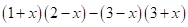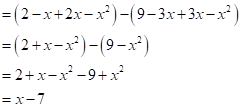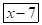# Perform the indicated operations and simplify.### Precalculus: Mathematics for Calcu...

6th Edition
Stewart + 5 others
Publisher: Cengage Learning
ISBN: 9780840068071### Precalculus: Mathematics for Calcu...

6th Edition
Stewart + 5 others
Publisher: Cengage Learning
ISBN: 9780840068071

#### Solutions

Chapter 1, Problem 51RE
To determine

## Perform the indicated operations and simplify.

Expert Solution### Explanation of Solution

Given information:

Perform the indicated operations and simplify.Calculation:

Consider the given expression.Solve the binomial,Hence, the resultant is### Have a homework question?

Subscribe to bartleby learn! Ask subject matter experts 30 homework questions each month. Plus, you’ll have access to millions of step-by-step textbook answers!